Published: 15 May 2016

# Bifurcation and chaos for electromechanical coupling torsional vibration in rolling mill system driven by DC motor

Haoran Liu1
Jinjie Liu2
Fei Liu3
Zhanlong Zhu4
Kun Wang5
Shuang Liu6
1, 3School of Information Science and Engineering, Yanshan University, Qinhuangdao, China
1, 3The Key Laboratory for Special Fiber and Fiber Sensor of Hebei Province, Yanshan University, Qinhuangdao, China
2, 4, 6School of Electrical Engineering, Yanshan University, Qinhuangdao, China
5School of Science, Yanshan University, Qinhuangdao, China
Corresponding Author:
Jinjie Liu
Views 86

#### Abstract

Bifurcation and chaos for electromechanical coupling torsional vibration in rolling mill system driven by DC motor is studied in this paper. Considering the electromechanical devices under unsaturated magnetic circuit, the dynamical equation of electromechanical coupling vibration in rolling mill drive system is deduced by using the dissipation Lagrange equation. The equivalent low-dimensional bifurcation equation, which can reveal the influence of system parameters on the nonlinear dynamic characteristics, is obtained by reducing the dimensionality system using the method of Lyapunov-Schmidt reduction, and the static bifurcation characteristic is analyzed by using the singularity theory. The torsional rigidity of rotating shaft and harmonic excitation are chosen as the bifurcation parameters. Finally, the simulation is carried out with actual parameters, the bifurcation diagram, Poincare map and the maximum Lyapunov exponent are also given, which show the influence of bifurcation parameters on the chaotic motions of the system.

## 1. Introduction

With the rapid development of the industrial technology, the rolling mill equipment continues melting towards maximization, high speed, automation and high accuracy directions. Torsional vibration of the rolling mill main drive system will serious affect the normal work and cause the damage to the equipment [1-3]. Torsional vibration is usually caused by nonlinear dynamic behaviors such as bifurcation and chaos. So there is great practice and immediate significance to study the nonlinear dynamic characteristics of the electromechanical coupling system.

The torsional vibration of rotational system was simulated and tested based on different work theory, which provides the experimental basis for torsional vibration analysis and control [4-6]. The low frequency torsional gap in shafts provides a new idea for vibration control, the vibration suppression in a lumped torsional system using wave-absorption control with online computation of an imaginary wave-propagation system was discussed, and the theoretical analysis showed that smaller mass and larger spring stiffness of an imaginary system absorb vibration energy better . The propagation of torsional wave in the shaft with periodically attached local resonators was studied and the effect of shaft material on the vibration attenuation in band gap was investigated in Ref. . Considering the effect of the backlash nonlinear factor of the relative rotation nonlinear dynamic system, the high-dimensional torsional vibration global dynamical equation was established based on Lagrange equation, the bifurcation characteristic was analyzed and the effects of the parameters on the vibration were discussed . Abstracting the rolling mill main drive system into two masses or multi-mass relative rotation system, the bifurcation and the chaos which may cause torsional vibration of system were studied [10, 11]. A nonlinear feedback controller was also proposed to control the Hopf bifurcation point and the stability of periodic solutions. Gustavsson R. K. et al.  analyzed the effects of unbalance magnetic pull on the transverse vibration characteristics of motor rotor system. Taking high frequency converting current of inverter power switches into consideration, the electromechanical coupling dynamic model of high speed grinding system was established, and the physical mechanism of higher harmonic electromechanical coupling vibration was revealed in Ref. . Wang Xingyuan et al. [14, 15] dealed with the existence of both Hopf bifurcation and topological horseshoe for a novel finance chaotic system. Kobayashi T. et al.  studied the effect of slot combination on acoustic noise from induction motors. Considering the high harmonic in electricity, Wu Huimin et al.  analyzed the effect of electromagnetic stiffness on the inherent frequency. Considering the influence of distribution of salient pole on air-gap permeance, model of electromechanical coupling torsional vibration for the rotor system under electromagnetic excitation was established by Xu Jinyou et al. . Taking the nonlinearity of the generator shaft and the interaction of mechanics and electrics in the generator sets into account, a transient model was obtained by combining Park equations and mechanics equations in et al. . The Hopf bifurcation, period-doubling bifurcation and chaos were investigated with nonlinear mode and Floquet theory. Considering the electromechanical devices with unsaturated magnetic circuit, the dynamic behavior of the system is analyzed by P. Woafo [20, 21].

Up to now, there are few researches about the electrical parameters in rolling mill torsional vibration system driven by DC motor. In this Letter, a transmission system driven by DC motor is abstracted as two masses torsional vibration model. The nonlinear electromechanical coupling dynamical equation of transmission system is established considering the coupling effect of electrical parameters and mechanical parameters. The equivalent low dimensional bifurcation equation is obtained and the bifurcation characteristic is analyzed by using the singularity theory. Finally, numerical simulations were performed to study the influence of torsional rigidity and excitation amplitude on chaotic motion. These provide a theoretical basis for design and control of the electromechanical coupling transmission system which is widely used in practical engineering.

## 2. Electromechanical coupling torsional vibration system

The main drive system of rolling mill usually consists of motor, universal couplings and rollers. The method of lumped mass is adopted to abstract the rolling mill main drive system driven by DC synchronous motor into a two-mass relative rotation model.

Considering the rolling mill main drive system under the incentive of torsional rigidity and outside disturbance, the total kinetic and potential energy of the system can be described as:

1
$E=\frac{1}{2}{J}_{1}{\theta }_{1}^{2}+\frac{1}{2}{J}_{2}{\theta }_{2}^{2},$
2
$U=\frac{1}{2}K\left({\theta }_{1}-{\theta }_{2}{\right)}^{2}.$

Damping force is:

3
${F}_{1}^{c}=-C\left({\stackrel{˙}{\theta }}_{1}-{\stackrel{˙}{\theta }}_{2}\right),$
4
${F}_{2}^{c}=-C\left({\stackrel{˙}{\theta }}_{2}-{\stackrel{˙}{\theta }}_{1}\right).$

Generalized torque is:

5
${Q}_{j}=\sum _{i=1}^{2}{F}_{i}^{i}\frac{\partial {\theta }_{i}}{\partial {q}_{j}},\mathrm{}\mathrm{}\mathrm{}\mathrm{}\left(j=1,\mathrm{}2\right),$

where ${q}_{j}$ ($j=$1, 2) is generalized coordinate. Substituting Eq. (3), Eq. (4) into Eq. (5), yields the generalized torque:

6
${Q}_{1}=\left({F}_{1}+{F}_{1}^{c}\right)\frac{\partial {\theta }_{1}}{\partial {\theta }_{1}}+\left({F}_{2}+{F}_{2}^{c}\right)\frac{\partial {\theta }_{2}}{\partial {\theta }_{1}}={F}_{1}-C\left({\stackrel{˙}{\theta }}_{1}-{\stackrel{˙}{\theta }}_{2}\right),$
7
${Q}_{2}=\left({F}_{1}+{F}_{1}^{c}\right)\frac{\partial {\theta }_{1}}{\partial {\theta }_{2}}+\left({F}_{2}+{F}_{2}^{c}\right)\frac{\partial {\theta }_{2}}{\partial {\theta }_{2}}={F}_{2}-C\left({\stackrel{˙}{\theta }}_{2}-{\stackrel{˙}{\theta }}_{1}\right).$

Substituting Eq. (6), Eq. (7) into Lagrange equation:

8
$\frac{d}{dt}\frac{\partial E}{\partial {\stackrel{˙}{\phi }}_{i}}-\frac{\partial E}{\partial {\phi }_{i}}+\frac{\partial U}{\partial {\stackrel{˙}{\phi }}_{i}}={Q}_{i},\mathrm{}\mathrm{}\mathrm{}\mathrm{}\left(i=1,\mathrm{}2\right).$

Yields:

9
$\left\{\begin{array}{l}{J}_{1}{\stackrel{¨}{\theta }}_{1}+K\left({\theta }_{1}-{\theta }_{2}\right)+C\left({\stackrel{˙}{\theta }}_{1}-{\stackrel{˙}{\theta }}_{2}\right)={F}_{1},\\ {J}_{2}{\stackrel{¨}{\theta }}_{2}-K\left({\theta }_{1}-{\theta }_{2}\right)-C\left({\stackrel{˙}{\theta }}_{1}-{\stackrel{˙}{\theta }}_{2}\right)={F}_{2},\end{array}\right\$

where ${J}_{1}$ and ${J}_{2}$ are inertia moments of the motor and mill roll, ${\theta }_{i}$ and ${\stackrel{˙}{\theta }}_{i}$ ($i=$1, 2) are rotational angle and the speed of the rotational angle of motor and mill roll, $K$ is linear torsional stiffness of the system, $C$ is structural damping of the system, ${F}_{1}$ is output torque, ${F}_{2}$ is the damping force.

During the functioning of the devices, nonlinearity appears in the components of the mechanical parts. Indeed, for hardening torsion or compression effects in mechanical problems, the stiffness is not constant but increases with the applied constraint. The coefficient of torsion or compression can be approximated by the relation:

10
$K={k}_{l}+{k}_{nl}{\left(\frac{{\theta }_{1}}{{\partial }_{0}}\right)}^{2}={k}_{l}\left[1+\delta {\left(\frac{{\theta }_{1}}{{\partial }_{0}}\right)}^{2}\right],$

where ${k}_{l}$ is the stiffness for small torsion or compression, ${\theta }_{1}$ is the rotation angle displacement, $\delta$ is the coefficient of nonlinearity and ${\partial }_{0}$ is the normalization angle, the motor has two parts: a stator or inductor in a permanent magnet and a rotor, mobile around a revolution axis. To avoid the Foucault effect and to decrease the hysteresis loss, the following assumptions are considered: symmetrical magnetic circuit, negligible magnetic hysteresis, negligible magnetic flux leak . Using Kirchhoff’s law, the equation of the electrical part is given by the following relation:

11
$u\left(t\right)=Ri+L\frac{di}{dt}+{K}_{E}\frac{d{\theta }_{1}}{dt},$

where, in the right, the first term is the ohmic voltage, the second term is the voltage across the inductor, and the third term ${K}_{E}d{\theta }_{1}/dt$ represents the coupling term between the electrical and mechanical parts. Using the Newton second law of dynamics for rotational motions and taking into account the Laplace force, the equation of the mechanical part is obtained as:

12
${J}_{\mathrm{\Delta }r}\frac{{d}^{2}{\theta }_{1}}{d{t}^{2}}=-{C}_{v}\frac{d{\theta }_{1}}{dt}+{K}_{T}i+{T}_{r},$

where ${J}_{\mathrm{\Delta }r}$ is the moment of inertia of the rotor, ${T}_{v}=-{C}_{v}d{\theta }_{1}/dt$ is the torque force due to viscous frictions, ${K}_{T}$ is the torque constant, ${K}_{T}i$ is the electromagnetic torque due to the Laplace force, ${T}_{r}$ is the resistive torque, it is modeled by the relation:

13
${T}_{r}=-K{\theta }_{1}=-{C}_{r}\left[1+\delta {\left(\frac{{\theta }_{1}}{{\partial }_{0}}\right)}^{2}\right]{\theta }_{1}={K}_{1}{\theta }_{1}+{K}_{2}{{\theta }_{1}}^{3}.$

When ${F}_{1}={K}_{T}i$ and ${F}_{2}={T}_{r}$ the system equations based on DC drive is:

14
$\left\{\begin{array}{l}{J}_{1}{\stackrel{¨}{\theta }}_{1}+K\left({\theta }_{1}-{\theta }_{2}\right)+C\left({\stackrel{˙}{\theta }}_{1}-{\stackrel{˙}{\theta }}_{2}\right)={K}_{T}i,\\ {J}_{2}{\stackrel{¨}{\theta }}_{2}-K\left({\theta }_{1}-{\theta }_{2}\right)-C\left({\stackrel{˙}{\theta }}_{1}-{\stackrel{˙}{\theta }}_{2}\right)={K}_{1}{\theta }_{1}+{K}_{2}{\theta }_{1}^{3},\\ Ri+L\frac{di}{dt}+{K}_{E}\frac{d{\theta }_{1}}{dt}=u\left(t\right).\end{array}\right\$

Eq. (14) is the nonlinear dynamic equation considering the torsional rigidity and the coupling term between the electrical and mechanical parts. In the process of motor running, ${K}_{1}$ is influenced by the motor load, the power supply voltage and the current. ${K}_{1}$ is selected as a major parameter to analyze the system dynamic characteristics in the following.

## 3. Bifurcation analysis of high dimensional electromechanical coupling system

If we want to analyze system characteristics the first step is dimensionless the data processing. In this paper, we use linear dimensionless method for data processing, assuming that the original data $x$ and dimensionless data $X$ have a linear relationship:

$X=xU,$

where $U$ is the dimensional scale, according to the practical parameters of electromechanical coupling torsional vibration system for 2150 rolling mill in a certain factory, a dimensionless form of linear proportional method is obtained by letting:

${t}^{\mathrm{*}}=\frac{\omega }{\mathrm{\Omega }}t,{x}_{1}=\frac{{\theta }_{1}}{{\theta }_{c}},{x}_{2}={\stackrel{˙}{x}}_{1},{x}_{3}=\frac{{\theta }_{2}}{{\theta }_{c}},{x}_{4}={\stackrel{˙}{x}}_{3},{x}_{5}=i,-\frac{K{\mathrm{\Omega }}^{2}}{{J}_{1}{\omega }^{2}}=a,-\frac{C\mathrm{\Omega }}{{J}_{1}\omega }=b,$
$\frac{K{\mathrm{\Omega }}^{2}}{{J}_{1}{\omega }^{2}}=c,\frac{C\mathrm{\Omega }}{{J}_{1}\omega }=d,\frac{{K}_{T}{\mathrm{\Omega }}^{2}}{{J}_{1}{\omega }^{2}}=e,\frac{\left(K+{K}_{1}\right){\mathrm{\Omega }}^{2}}{{J}_{2}{\omega }^{2}}=g,\frac{C\mathrm{\Omega }}{{J}_{2}\omega }=h,-\frac{K{\mathrm{\Omega }}^{2}}{{J}_{2}{\omega }^{2}}=m,-\frac{C\mathrm{\Omega }}{{J}_{2}\omega }=n,$
$\frac{{K}_{2}{\theta }_{c}^{2}{\mathrm{\Omega }}^{2}}{{J}_{2}{\omega }^{2}}=p,-\frac{{K}_{E}\mathrm{\Omega }}{L\omega }=s,-\frac{R\mathrm{\Omega }}{L\omega }=z,T=\frac{u\left(t\right)}{L},$

where $\omega /\mathrm{\Omega }$ is system dimensionless ratio value, ${\theta }_{c}$ is standard reduced value for the system angle.

Considering the excitation amplitude of $F$ is added at the load equipment, Then Eq. (14) can be reduced order for first-order equation as follows:

15
$\left\{\begin{array}{l}{\stackrel{˙}{x}}_{1}={x}_{2},\\ {\stackrel{˙}{x}}_{2}=a{x}_{1}+b{x}_{2}+c{x}_{3}+d{x}_{4}+e{x}_{5},\\ {\stackrel{˙}{x}}_{3}={x}_{4},\\ {x}_{4}=g{x}_{1}+h{x}_{2}+m{x}_{3}+n{x}_{4}+p{x}_{1}^{3}+F,\\ {\stackrel{˙}{x}}_{5}=s{x}_{2}+z{x}_{5}.\end{array}\right\$

### 3.1. Lyapunov Schmidt reduction

Eq. (15) can be expressed as:

16
$\stackrel{˙}{X}=f\left(X\right),$

where $X=\left({x}_{1},{x}_{2},{x}_{3},{x}_{4},{x}_{5}{\right)}^{T}$ is the state variables of dynamic system and $f\left(X\right)=\left({f}_{1},{f}_{2},{f}_{3},{f}_{4},{f}_{5}{\right)}^{T}$ are the corresponding nonlinear vector functions.

At the equilibrium point $\stackrel{-}{X}$, system Eq. (16) satisfies:

17
$f\left(\stackrel{-}{X}\right)=0.$

Let $Df\left(\stackrel{-}{X}\right)$ be the Jacobian matrix of $f\left(X\right)$ at the equilibrium:

18
$Df\left(\stackrel{-}{X}\right)=\left(\begin{array}{lllll}\frac{\partial {f}_{1}}{\partial {x}_{1}}& \frac{\partial {f}_{1}}{\partial {x}_{2}}& \frac{\partial {f}_{1}}{\partial {x}_{3}}& \frac{\partial {f}_{1}}{\partial {x}_{4}}& \frac{\partial {f}_{1}}{\partial {x}_{5}}\\ \frac{\partial {f}_{2}}{\partial {x}_{1}}& \frac{\partial {f}_{2}}{\partial {x}_{2}}& \frac{\partial {f}_{2}}{\partial {x}_{3}}& \frac{\partial {f}_{2}}{\partial {x}_{4}}& \frac{\partial {f}_{2}}{\partial {x}_{5}}\\ \frac{\partial {f}_{3}}{\partial {x}_{1}}& \frac{\partial {f}_{3}}{\partial {x}_{2}}& \frac{\partial {f}_{3}}{\partial {x}_{3}}& \frac{\partial {f}_{3}}{\partial {x}_{4}}& \frac{\partial {f}_{3}}{\partial {x}_{5}}\\ \frac{\partial {f}_{4}}{\partial {x}_{1}}& \frac{\partial {f}_{4}}{\partial {x}_{2}}& \frac{\partial {f}_{4}}{\partial {x}_{3}}& \frac{\partial {f}_{4}}{\partial {x}_{4}}& \frac{\partial {f}_{4}}{\partial {x}_{5}}\\ \frac{\partial {f}_{5}}{\partial {x}_{1}}& \frac{\partial {f}_{5}}{\partial {x}_{2}}& \frac{\partial {f}_{5}}{\partial {x}_{3}}& \frac{\partial {f}_{5}}{\partial {x}_{4}}& \frac{\partial {f}_{5}}{\partial {x}_{5}}\end{array}\right).$

The Lyapunov stability is decided by the eigenvalues of $Df\left(\stackrel{-}{X}\right)$. The Jacobian matrix $Df\left(\stackrel{-}{X}\right)$ can be expressed as:

19
$Df\left(\stackrel{-}{X}\right)=\left(\begin{array}{ccccc}0& 1& 0& 0& 0\\ a& b& c& d& e\\ 0& 0& 0& 1& 0\\ g+3p{x}_{1}^{2}& h& m& n& 0\\ 0& s& 0& 0& z\end{array}\right).$

This is a 5×5 square matrix and the value of the determinant is:

20
$\mathrm{d}\mathrm{e}\mathrm{t}\left(L\right)={\lambda }^{5}+\left(-n-z-b\right){\lambda }^{4}+\left(nz+bz+bn-hd-se-a-m\right){\lambda }^{3}$
$\mathrm{}\mathrm{}\mathrm{}\mathrm{}\mathrm{}\mathrm{}+\left(hdz+an-bnz+az+bm+mz+sen-hc-gd-3pd{x}_{1}^{2}\right){\lambda }^{2}$
$\mathrm{}\mathrm{}\mathrm{}\mathrm{}\mathrm{}\mathrm{}+\left(am+h\mathrm{c}z-anz-bmz+sme-\mathrm{g}c+gdz-3pc{x}_{1}^{2}+3pdz{x}_{1}^{2}\right)\lambda$
$\mathrm{}\mathrm{}\mathrm{}\mathrm{}\mathrm{}\mathrm{}+gcz+3pcz{x}_{1}^{2}-amz=0.$

There is a zero eigenvalue when $gcz+3pcz{x}_{1}^{2}-amz=0$, and $L$ is a singular matrix.

It can be known that $\mathrm{d}\mathrm{i}\mathrm{m}N\left(L\right)=$ 1, let ${v}_{0}$ be a basis vector of null space $N\left(L\right)$, then we can get ${v}_{0}=\left(-c/a,0,1,0,0\right)$. The adjoint operator of $L$ is the conjugate transpose matrix ${L}^{\mathrm{*}}$. Because $L$ is a real matrix and the conjugate transpose matrix ${L}^{\mathrm{*}}$ is also a real matrix, and there is ${L}^{\mathrm{*}}={L}^{T}$. We can see that $\mathrm{d}\mathrm{i}\mathrm{m}N\left({L}^{*}\right)=\mathrm{}$1 since $L$ is a Fredholm operator with zero index. One basis vector:

${v}_{1}=\left(\frac{bq}{a}-\frac{qse}{az}-h,-\frac{q}{a},\frac{dq}{a}-n,1,-\frac{eq}{az}\right),$

of $\mathrm{d}\mathrm{i}\mathrm{m}N\left({L}^{*}\right)=\mathrm{}$1 is obtained by calculation, where $q=g+3p{{x}_{1}}^{2}$. So the five-dimensional Euclid space ${R}^{5}$ can be decomposed as follows:

${R}^{5}=N\left(L\right)\oplus M=R\left(L\right)\oplus N\left({L}^{\mathrm{*}}\right).$

Define the projection operator:

$P:{R}^{5}\to R\left(L\right),$

and the complementary projection operator:

$I-P:{R}^{5}\to N\left({L}^{\mathrm{*}}\right)=\mathrm{s}\mathrm{p}\mathrm{a}\mathrm{n}\left\{{v}_{1}\right\},$

then the equation:

21
$f\left(x,p\right)=0,$

equivalent to:

22
$\left\{\begin{array}{l}Pf\left(v+w,p\right)=0,\\ \left(I-P\right)f\left(v+w,p\right)=0,\end{array}\right\$

where $v\in N\left(L\right)=\mathrm{s}\mathrm{p}\mathrm{a}\mathrm{n}\left({v}_{0}\right)$, $w\in M$. According to the definition of inner product in Euclid space, $\left(I-P\right)f\left(v+w,p\right)=0$ is equivalent to:

23
$〈f\left(v+w,p\right),{v}_{1}〉=0.$

Thus, the following equation is obtained:

24
$\left(g+a\right)\left(\frac{bq}{a}-\frac{esq}{az}-h\right)+p{\left(\frac{bq}{a}-\frac{esq}{az}-h\right)}^{3}-\frac{q}{a}\left(1+b+h+s\right)$
$\mathrm{}\mathrm{}\mathrm{}\mathrm{}\mathrm{}\mathrm{}\mathrm{}+\left(c+m\right)\left(\frac{dq}{a}-n\right)+\frac{eq}{az}\left(e+z\right)+1+d+n+F=0.$

Since $gcz+3pcz{x}_{1}^{2}-amz=0$, rewritten this equation and substitute it into Eq. (24), then the expression of ${x}_{1}$ can be got:

25
$A{x}_{1}^{6}+B{x}_{1}^{4}+C{x}_{1}^{2}+D=0,$

where:

$A=p{\left(\frac{3bp}{a}-\frac{3pse}{az}\right)}^{3},B=3p\left(\frac{bg}{a}-\frac{seg}{az}-h\right){\left(\frac{3bp}{a}-\frac{3pse}{az}\right)}^{2}-3p\left(\frac{3bp}{a}-\frac{3pse}{az}\right),$
$C=\frac{3pd\left(c+m\right)}{a}+3p\left(\frac{3bp}{a}-\frac{3pse}{az}\right){\left(\frac{bg}{a}-\frac{seg}{az}-h\right)}^{2}+\left(a+\frac{am}{c}\right)\left(\frac{3bp}{a}-\frac{3pse}{az}\right)$
$\mathrm{}\mathrm{}\mathrm{}\mathrm{}\mathrm{}-3p\left(\frac{bg}{a}-\frac{seg}{az}-h\right)-3\frac{p}{a}\left(1+b+h+s\right)+\frac{3pe\left(e+z\right)}{az},$
$D=\left(\frac{am}{c}+a\right)\left(\frac{bg}{a}-\frac{seg}{az}-h\right)+p{\left(\frac{bg}{a}-\frac{seg}{az}-h\right)}^{3}+\left(c+m\right)\left(\frac{dg}{a}-n\right)$
$\mathrm{}\mathrm{}\mathrm{}\mathrm{}\mathrm{}-\frac{g\left(1+b+h+s\right)}{a}+\frac{eg\left(e+z\right)}{az}+d+n+1+F.$

Assuming that a new state variable is $r={x}_{1}$, then the bifurcation equation can be written as:

26
$G={r}^{6}+{\alpha }_{1}{r}^{4}+{\alpha }_{2}{r}^{2}+\beta ,$

where ${\alpha }_{1}$, ${\alpha }_{2}$ as the open fold parameters of bifurcation equation are both for the physical parameters of the system.

### 3.2. Singularity analysis

The topology of Eq. (26) and the effects of the open fold parameters on the bifurcation behavior will be studied by using the singularity theory. The following point sets are obtained based on the definition of the transition set:

I: Bifurcation point set:

$B=\left\{\left({\alpha }_{1},{\alpha }_{2}\right)|G={G}_{r}={G}_{\beta }=0\right\}=\mathrm{\Phi }.$

II: Lag point sets:

${H}_{1}=\left\{\left(\left({\alpha }_{1},{\alpha }_{2}\right)\right)|G={G}_{r}={G}_{rr}=0\right\}=\left\{\left({\alpha }_{1},{\alpha }_{2}\right)|{\alpha }_{2}=0\right\},$
${H}_{2}=\left\{\left(\left({\alpha }_{1},{\alpha }_{2}\right)\right)|G={G}_{r}={G}_{rr}=0\right\}=\left\{\left({\alpha }_{1},{\alpha }_{2}\right)|{\alpha }_{2}=\frac{{\alpha }_{1}^{2}}{3},{\alpha }_{1}\le 0\right\}.$

III: Bilimits point set:

$D=\left\{\left(\left({\alpha }_{1},{\alpha }_{2}\right)\right)|G={G}_{r}=0\right\}=\left\{\left({\alpha }_{1},{\alpha }_{2}\right)|{\alpha }_{2}=\frac{{\alpha }_{1}^{2}}{4},{\alpha }_{1}\le 0\right\}.$

IV: Transition set:

$\sum ={H}_{1}\cup {H}_{2}\cup D.$

Transition set $\sum$ is shown in Fig. 1, the unfolding parametric space ${\alpha }_{1}-{\alpha }_{2}$ is divided into four subregions (I, II, III, IV) by $\sum$ and Eqs. (5)-(9) are bifurcation response curves of $\sum$. Each subregion and its boundary bifurcation are shown in Fig. 2, Through the analysis of Fig. 2 we can draw the conclusion that: the roots of the Eq. (26) are smooth distribution in subregion I, the roots of the Eq. (26) have amplitude jump in subregion 2-9, This means that the system is not stable and such situations should be avoided in system designing and controlling.

Fig. 1Transition set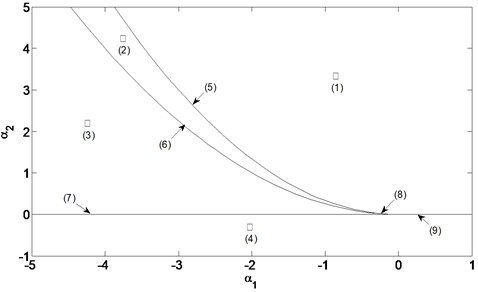## 4. Numerical simulations of chaotic processes

As harmonic excitation often occurs torsional vibration in the process of the motor operation, and the harmonic excitation is not a fixed value, but cyclical changes over time, by Fourier transform it generally expressed as a linear combination of trigonometric functions (sine/cosine) or their integration. This paper adopts $F=f\mathrm{c}\mathrm{o}\mathrm{s}\left(wt\right)$ for harmonic excitation, it is the general expression. In order to study the dynamic chaos of electromechanical coupling system, Runge-Kutta method is used for numerical simulation of torsion vibration system. Then Eq. (15) can be expressed as:

27
$\left\{\begin{array}{l}{\stackrel{˙}{x}}_{1}={x}_{2},\\ {\stackrel{˙}{x}}_{2}=a{x}_{1}+b{x}_{2}+c{x}_{3}+d{x}_{4}+e{x}_{5},\\ {\stackrel{˙}{x}}_{3}={x}_{4},\\ {x}_{4}=g{x}_{1}+h{x}_{2}+m{x}_{3}+n{x}_{4}+p{x}_{1}^{3}+f\mathrm{c}\mathrm{o}\mathrm{s}\left(wt\right),\\ {\stackrel{˙}{x}}_{5}=s{x}_{2}+z{x}_{5}.\end{array}\right\$

Fig. 2Bifurcation diagram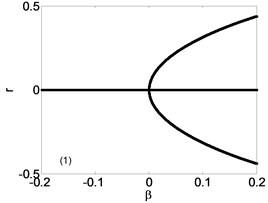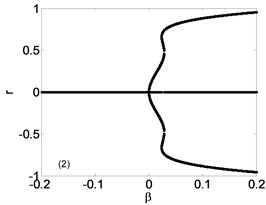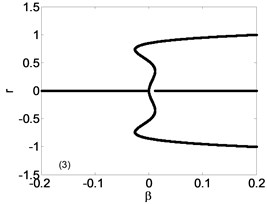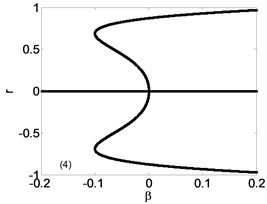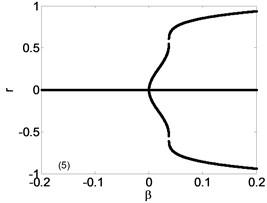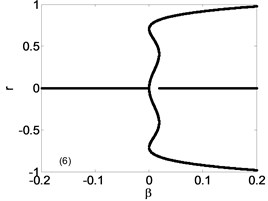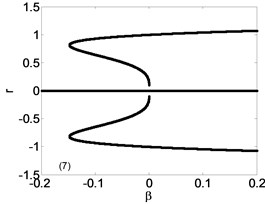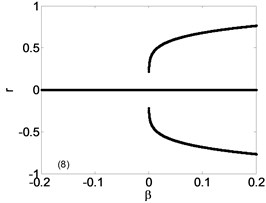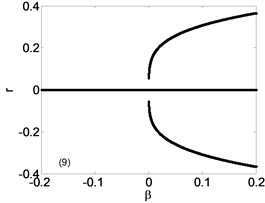Fig. 3Bifurcation diagram in K1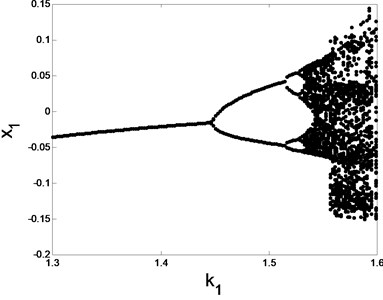Fig. 4The maximum Lyapunov exponentThe system parameters can be taken as $a=$ –1.5, $b=\mathrm{}$–0.2, $c=$ 1.5, $d=$ 0.2, $e=$ 1.4, $h=$ 2, $m=$ –15, $n=$ –2, $p=$ –22, $s=$ –1.5, $z=$ –0.575, $w=$ 1 through dimensionless. The influence of torsional rigidity ${K}_{1}$ and excitation amplitude $f$ on electromechanical coupling system is discussed separately in this section. Take $f=\mathrm{}$1.54 and with other parameters constant, while ${K}_{1}$ change in a wide range. The bifurcation diagrams and the maximum Lyapunov exponent of ${K}_{1}$ are shown in Fig. 3 and Fig. 4. It shows that system jumps into chaotic motion from a stable periodic motion with the increase of ${K}_{1}$.

Fig. 5Phase trajectory (–1), Poincare map (–2) for different K1: a) K1= 1.4: b) K1= 1.5, c) K1= 1.52, d) K1= 1.58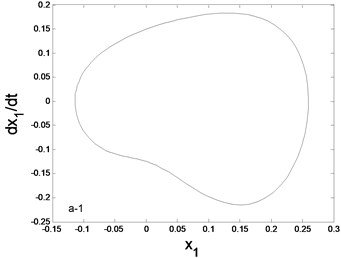a1) Phase trajectory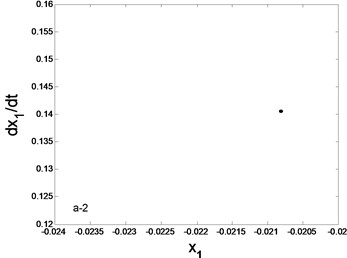a2) Poincare map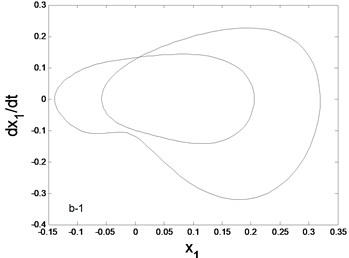b1) Phase trajectory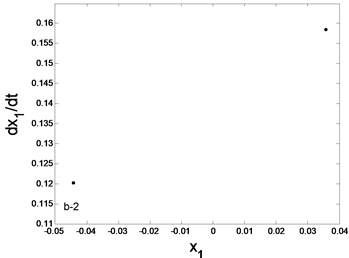b2) Poincare map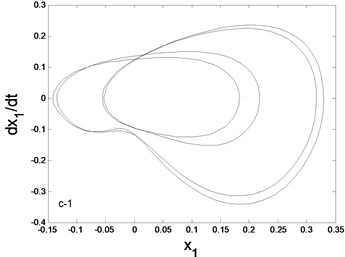c1) Phase trajectory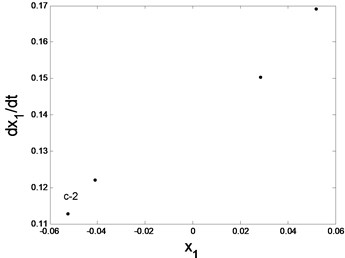c2) Poincare map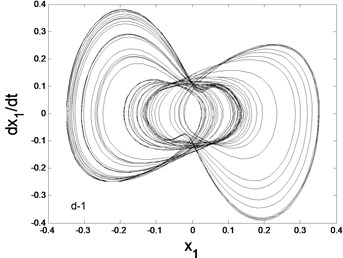d1) Phase trajectory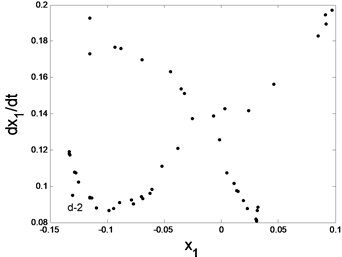d2) Poincare map

The system phase diagrams and Poincare maps diagram under different ${K}_{1}$ are shown in Fig. 5. From Fig. 3, we can see that the bifurcation diagram changed when ${K}_{1}=\mathrm{}$1.4, ${K}_{1}=$ 1.5 and ${K}_{1}=$ 1.52, system response is a stable periodic motion. It can be seen from Fig. 5(a)-(c) that the phase trajectory is a closed curve and the Poincare map has finite fixed points. From Fig. 4, the maximum Lyapunov exponent is greater than zero when ${K}_{1}=\mathrm{}$1.58, it can be convincing of occurrence of chaotic motion. We can also see that phase trajectory repeatedly winding in enclosed area but not closed, and Poincare map has the obvious fractal structure from Fig. 5(c1), (c2).

When ${K}_{1}=\mathrm{}$1.56 and keeps the other parameters constant, let $f$ change in a wide range. From the bifurcation diagram (Fig. 6.) and the maximum Lyapunov exponent (Fig. 7.) of $f$, it can be seen that system jumps into single periodic motion from chaotic motion with the increase of $f$.

Fig. 6Bifurcation diagram in f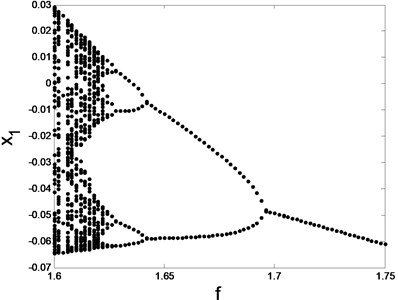Fig. 7The maximum Lyapunov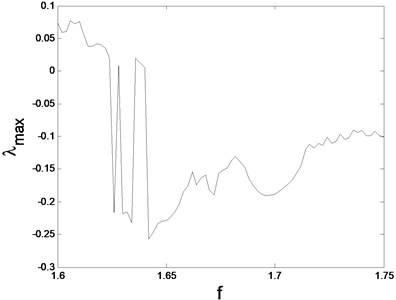The system phase diagrams and Poincare maps with different $f$ are shown in Fig. 8 From Fig. 7, the maximum Lyapunov exponent is greater than zero when $f=\text{1.6}$, and the system appears chaotic motion. We can also see that phase trajectory repeatedly winding in enclosed area but not closed, and the Poincare map has the obvious fractal structure from Fig. 8(a). From Fig. 7, the maximum Lyapunov exponent is lower than zero when $f=\mathrm{}$1.63, $f=\mathrm{}$1.65 and $f=\mathrm{}$1.7, system response appears a stable periodic motion. Then Fig. 8(b)-(d) show that phase trajectory is a closed curve, and Poincare map for a finite number of fixed points.

According to the numerical simulation, we come to a conclusion that both reducing torsional rigidity and increasing excitation amplitude can control the system from chaotic motion into stable periodic motion.

In order to control the chaotic motion, the external torque $T$ is introduced, assuming that kinetic equation with external torque $T$ is:

${\stackrel{˙}{x}}_{i}\left(t\right)={f}_{i}\left(x,t\right)+T.$

The original chaotic motion will be changed and the new stable periodic motion will appear when we adjust the external torque $T$. The external torque $T$ must be kept in non-zero value after the system movement is driven to the stable periodic motion, at this moment we can achieve the goal of chaos control. The value range of $T$ can be determined according to the Lyapunov stability theory and adjusting the Lyapunov index size.

In this paper the external torque $T$ is joined when the system appears chaotic motion, and we can select the appropriate torque force to control chaotic motion through analyzing the system bifurcation diagram and the maximum Lyapunov exponent when the torque changes. This method does not need to know the stable periodic orbit and state variables of the system. It only needs to load the system’s motion to the periodic motion, by using the external torque, this method is simple and has little influence on the system's motor parameters.

Fig. 8Phase trajectory (–1), Poincare map (–2) for different f: a) f= 1.6, b) f=1.63, c) f=1.65, d) f= 1.7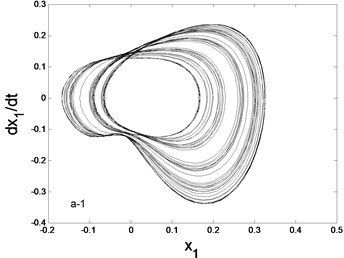a1) Phase trajectory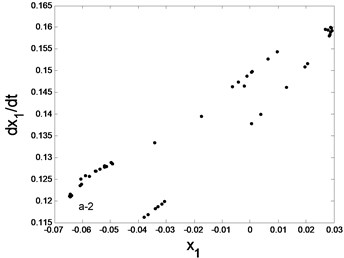a2) Poincare map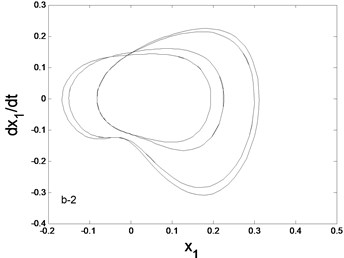b1) Phase trajectory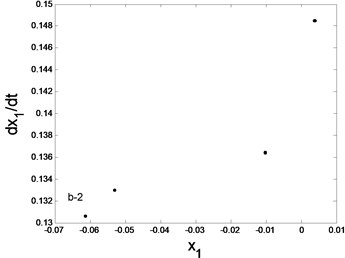b2) Poincare map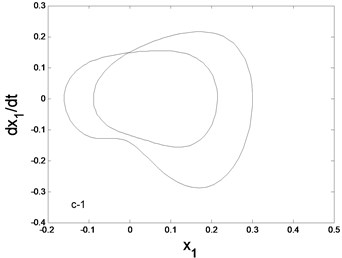c1) Phase trajectory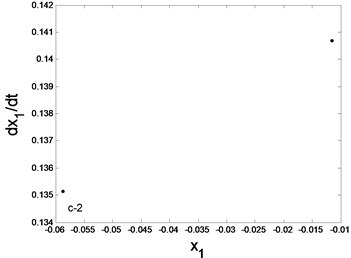c2) Poincare map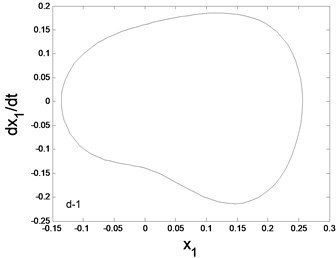d1) Phase trajectory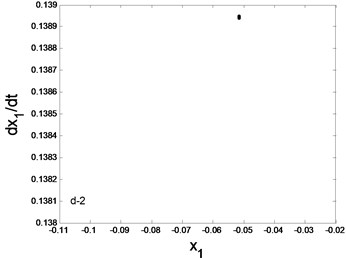d2) Poincare map

## 5. Conclusions

In this paper, considering the electromechanical devices with unsaturated magnetic circuit, a high dimensional dynamical equation of electromechanical coupling vibration in rolling mill drive system are deduced by using the dissipation Lagrange equation. The equivalent low-dimensional bifurcation equation which can reveal the influence of system parameters on the nonlinear dynamic characteristics is obtained by reducing the dimensionality of the system. The static bifurcation is studied by using the singularity theory. Considering outside incentive, choose ${K}_{1}$ and $f$ are chosen as bifurcation parameters, Numerical simulations are also given at last, which show that both reducing torsional rigidity and increasing excitation amplitude can control the system from chaotic motion into stable periodic motion, we can also add a constant torque direct feedback method to control chaos. These results have an important theoretical significance on reducing the vibration of electromechanical coupling transmission system. In addition, the research in our paper provides a reference for the real design of motor’s parameters of electromechanical coupling transmission system.

#### Cited by

Influence Factors of Inherent Characteristics of F5 Cold-Rolling Mill’s Main Drive System

18 October 2015
Accepted
18 January 2016
Published
15 May 2016
SUBJECTS
Chaos, nonlinear dynamics and applications
Keywords
electromechanical coupling
Lyapunov-Schmidt method
Lyapunov exponent
chaos
Acknowledgements

Project supported by the Natural Science Foundation of Hebei Province, China (Grant No. E2015203349).

Author Contributions

Contribution of each individual co-author to this article. Jinjie Liu – the dynamic model of the electromechanical coupling transmission system driven by DC motor is established, and the bifurcation characteristics of the system are analyzed by using the method of L-S reduction. Fei Liu – chaotic motion analysis of electromechanical coupling transmission system. Zhanlong Zhu – acquired data, and played an important role in interpreting the results. Kun Wang – helped perform the analysis with constructive discussions. Shuang Liu – revised the manuscript and approved the final version.